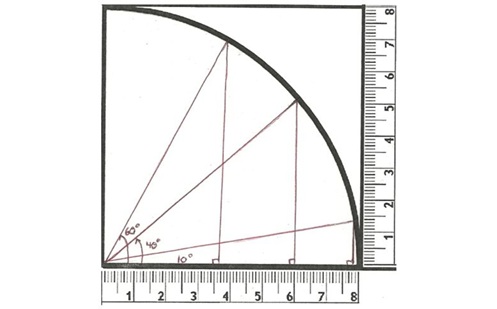# Introduction to trigonometry

These lesson plans guide the teacher on how to introduce trigonometry to students through an investigation of similar triangles.

Year level(s) Year 9
Audience Teacher
Format Web page
Teaching strategies and pedagogical approaches Mathematics investigation
Keywords Pythagoras, Hypotenuse, Adjacent, Opposite, Tangent, Cosine, Sine, Angle, Ratio, Similar, Trigonometry, Right-angled triangle

## Curriculum alignment

Curriculum connections Critical and creative thinking, Numeracy
Strand and focus Space
Topics Pythagoras and trigonometry
AC: Mathematics (V9.0) content descriptions
AC9M9SP02
Apply the enlargement transformation to shapes and objects using dynamic geometry software as appropriate; identify and explain aspects that remain the same and those that change

AC9M9M03

Solve spatial problems, applying angle properties, scale, similarity, Pythagoras' theorem and trigonometry in right-angled triangles

Numeracy progression Proportional thinking (P7)
Understanding geometric properties (P7)
Understanding units of measurement (P10)